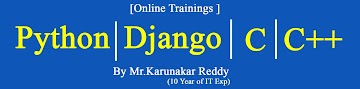-->
Home ---- ------ Contact### Microsoft-Excel 2007 Study Material Day-twenty three

Logical Functions:

1) AND ( ) : Returns true if all arguments are true ,returns false if one or more arguments is false.
Syntax : =AND(condition)

Example :

2) OR ( ) : Returns true if any arguments is true ,return false if all arguments are false.
Syntax : =OR(Condition)
Example :

3) NOT ( ) : Use the NOT function, one of the logical functions, when you want to make sure one value is not equal to another.
Syntax : =NOT(Condition)
Example :

4) IF ( ) : The IF function is one of the most popular functions in Excel, and it allows you to make logical comparisons between a value and what you expect.
Syntax : =if(logical_test,[value_if_true],[value_if_false])

Example :

Total      :   =SUM(C2:E2)

Average :   =AVERAGE(C2:E2)

Result    :  =IF(AND(C2>=35,D2>=35,E2>=35),"PASS","FAIL")